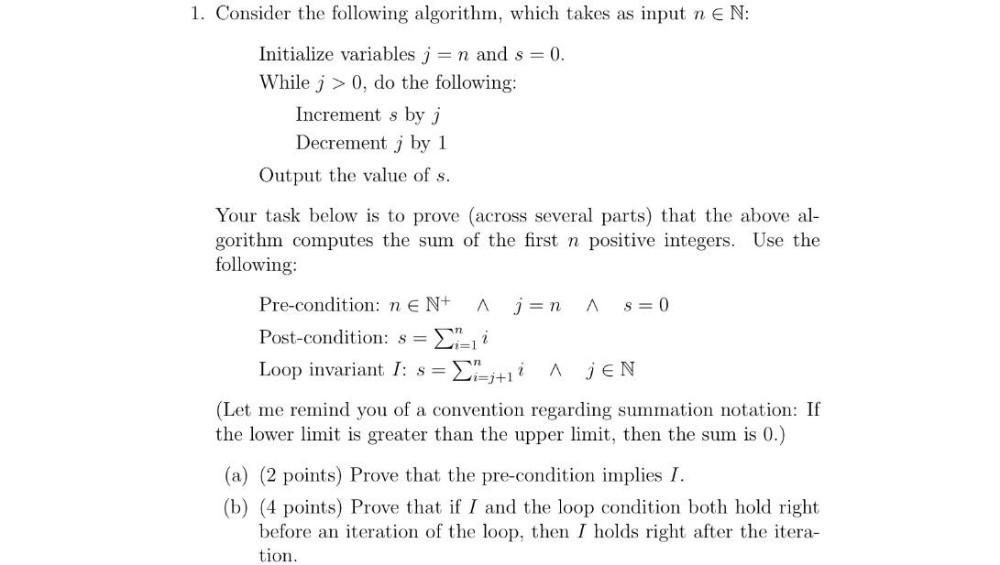Question:

# 1. Consider the following algorithm, which takes as input n EN: Initialize variables j = n and s = 0. While j > 0, do the follow1. Consider the following algorithm, which takes as input n EN: Initialize variables j = n and s = 0. While j > 0, do the following: Increment s by i Decrement j by 1 Output the value of s. Your task below is to prove (across several parts) that the above al- gorithm computes the sum of the first n positive integers. Use the following: A Pre-condition: ne N+ ján A s=0 Post-condition: s= i Loop invariant I: s= = {i=j+11 A MEN (Let me remind you of a convention regarding summation notation: If the lower limit is greater than the upper limit, then the sum is 0.) (a) (2 points) Prove that the pre-condition implies I. (b) (4 points) Prove that if I and the loop condition both hold right before an iteration of the loop, then I holds right after the itera- tion.